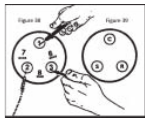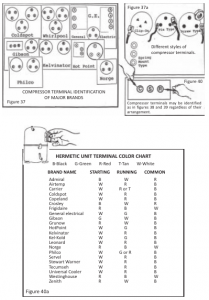# HOW TO IDENTIFY UNMARKED COMPRESSOR TERMINALS

Occasionally you will encounter some compressor terminals which have no markings (or which may have been obliterated). There is an easy way to determine the compressor C, S, and R terminals. Here is how:

Set the ohmmeter on its lowest scale. In figure 38, imagine the unmarked Set the ohmmeter on its lowest scale. In figurterminals as 1, 2, and 3. Place the two ohmmeter probes on terminals 1 and 2; make a note of the reading (7 Ω, for instance). Then place the probes on terminals 2 and 3 (assume 8 Ω, and note it).Finally, take a reading between 1 and 3 (assume the meter indicates 6 Ω). The highest reading between any two terminals means that the remaining one is the common terminal.

Since number 2 and number 3 terminals have the highest reading, it can be deduced that number 1 has to be the common terminal.

Since it has already been noted that the reading between terminals 1 and 2 is 7 Ω and the reading between terminals 1 and 3 is 6 Ω, the highest of these last two readings determines the start terminal. In other words, number 2 is the start terminal, so the remaining terminal (number 3) will have to be the run terminal (see fig. 39). Some terminal configurations appear as in figure 40. Use the same method to identify these too.